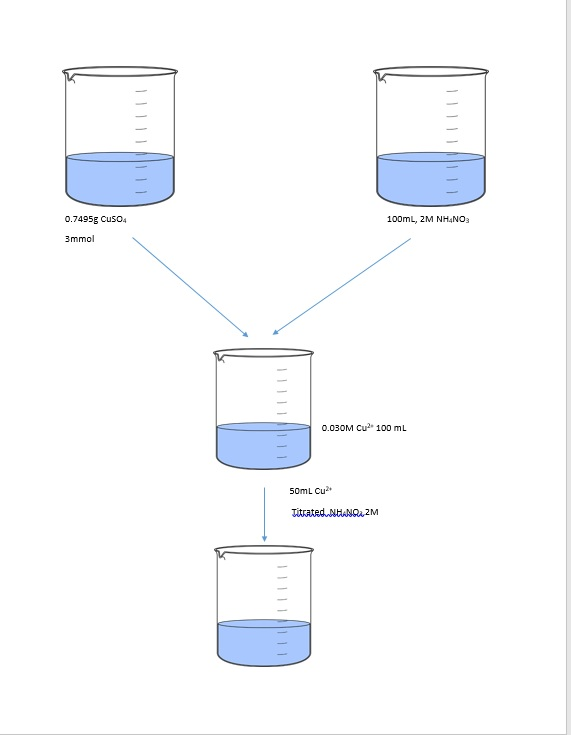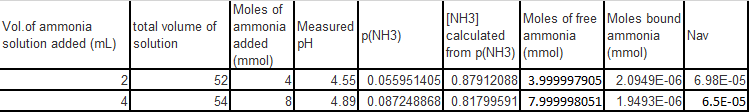# Question & Answer: It is given that, [NH3]0 [H+]0 = [NH3]x [H+]x (equation 1) where subscript 0 refers to the…..

It is given that, [NH3]0 [H+]0 = [NH3]x [H+]x (equation 1) where subscript 0 refers to the initial values in a stock solution of NH3, and the subscript x refers to the values in an unknown mixture (i.e during titration) both in 2M NH4NO3. Since pX is defined as the negative log10 of X, equation (1) can be written as:

p(NH3)0 + pH0 = p(NH3)x + pHx (equation 2)

Don't use plagiarized sources. Get Your Custom Essay on
Question & Answer: It is given that, [NH3]0 [H+]0 = [NH3]x [H+]x (equation 1) where subscript 0 refers to the…..
GET AN ESSAY WRITTEN FOR YOU FROM AS LOW AS \$13/PAGE

p(NH3)x = p(NH3)0 + pH0 – pHx (equation 3) where pHx is the measured pH at any time during titration. Thus, concentration of free NH3 can be calculated directly by applying equation 3. The total Cu2+ in solution at any point is defined as ccu where

ccu = [Cu2+] + [Cu(NH3)2+] [Cu(NH3)22+] + [Cu(NH3)32+] + [Cu(NH3)42+​]

The fractions, an of each species present at any point can be found by using the expressions for the stepwise formation constants.

a0 = [M]/ccu = (1+ K1[NH3] + K1K2[NH3]2 + K1K2K3[NH3]3)-1

a1 = [Cu(NH3)2+] / ccu = K1[NH3]a0

a2 = [Cu(NH3)22+] / ccu = K1K2[NH3]2a0

The average number of NH3 bound per Cu2+ or the ligand number (nav) can be calculated:

nav = [NH3]bound / ccu = ([NH3]total – [NH3]free) / ccu = a1+2a2+3a3+4a4

In the experiment, the 0.7495 g (3 mmol) copper sulfate was dissolved in 100mL 2M NH4NO3 solution to form 0.030 M Cu2+ solution (100 mL). 50 mL of copper solution was transferred to 200 mL beaker and titrated with 2M NH4NO3 solution from a 50mL burette in 2 mL aliquots. The pH was measured after each addition. Given the information, calculate

 Vol.of ammonia solution added total volume of solution Moles of ammonia added (mmol) Measured pH p(NH3) [NH3] calculated from p(NH3) Moles of free ammonia (mmol) Moles bound ammonia (mmol) Nav 2.00mL 52mL 4.55 4.00 mL 54mL 4.89

Calculate Moles of ammonia added (mmol), p(NH3), [NH3] calculated from p(NH3), Moles of free ammonia (mmol), Moles bound ammonia (mmol), Nav

If the Moles of ammonia added (mmol) cannot be calculated, pretend that 1st value of moles of ammonia added was 0.384 mmol.

Thank you very muchTo know what is the volume of ammonia added we know that the initial concentration is 2M [mmol/mL]

p(NH3)x = p(NH3)0 + pH0 – pHx to stimate P(NH3)x

(NH3)= 10^(-p(NH3)x)

ccu = [Cu2+] + [Cu(NH3)2+] [Cu(NH3)22+] + [Cu(NH3)32+] + [Cu(NH3)42+​]

a0 = [M]/ccu = (1+ K1[NH3] + K1K2[NH3]2 + K1K2K3[NH3]3)-1

a1 = [Cu(NH3)2+] / ccu = K1[NH3]a0

a2 = [Cu(NH3)22+] / ccu = K1K2[NH3]2a0

nav = [NH3]bound / ccu = ([NH3]total – [NH3]free) / ccu = a1+2a2+3a3+4a4